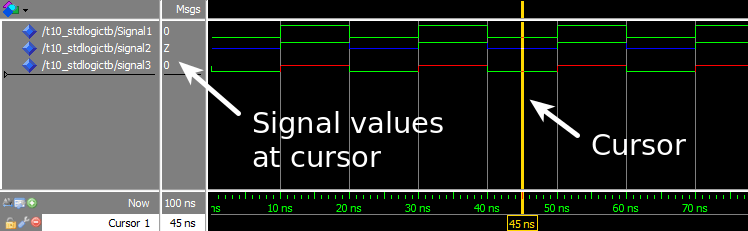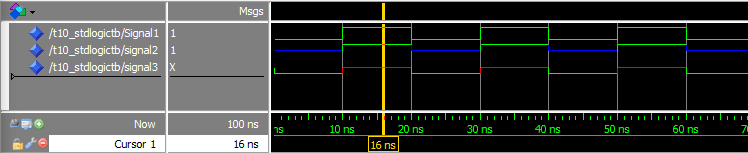# چطوری از STD_logic استفاده کنیم.

چطوری از STD_logic  استفاده کنیم.

The most common type used in VHDL is the `std_logic`. Think of this type as a single bit, the digital information carried by a single physical wire. The `std_logic` gives us a more fine-grained control over the resources in our design than the `integer` type, which we have been using in the previous tutorials.

Normally, we want a wire in a digital interface to have either the value `'1'` or `'0'`. These two values are the only values that a bit, a binary digit, can have. But in reality, a physical digital signal can be in a number of states, which the `std_logic` type does a good job emulating. Therefore it is the most frequently used type in VHDL.

The `std_logic` type can have the following values:

This may seem like a lot of different states for a type that is supposed to model a single binary value. Don’t worry, we won’t be using all these types in this tutorial series. We will be using `'1'` and `'0'` of course. And we will also be seeing `'U'` and `'X'`, which will help us spot errors in our design. The other values are advanced VHDL features which can be used for things like modeling communication with for example I2C devices, or for creating tri-state buses.

If several processes are trying to write different values to a signal, we say that it has multiple drivers. If a `std_logic` signal has multiple drivers, it won’t be a compilation or run-time error, at least not in the simulator. That is because `std_logic` is a resolved type, meaning that its value will be determined by a resolution function.

The value of a `std_logic` signal with two drivers will be determined based on this resolution table:

 ‘1’ Logic 1 ‘0’ Logic 0 ‘Z’ High impedance ‘W’ Weak signal, can’t tell if 0 or 1 ‘L’ Weak 0, pulldown ‘H’ Weak 1, pullup ‘-‘ Don’t care ‘U’ Uninitialized ‘X’ Unknown, multiple drivers

The waveform window in ModelSim after we pressed run, and zoomed in on the timeline:The waveform with the cursor placed on the other part of the repeating signal cycleThe waveform with the cursor placed on the other part of the repeating signal cycle:

The exercise demonstrated how the resolution function of VHDL works with the `std_logic` type. When working with digital logic it’s often more practical to study the timeline in a waveform rather than using printouts. Therefore we used the ModelSim waveform to check the signal values in this exercise.

The first process and `Signal1` is only used for changing the value that the third process is driving on `Signal2` and `Signal3`.

The second process, Driver A, will try to drive a `'Z'` onto `Signal2`, and a `'0'` onto `Signal3` constantly.

The third process, Driver B, will alternate between driving `'1'` and `'Z'` onto both `Signal2` and `Signal3`.

We see in the waveform screenshots that `Signal1` is changing between `'0'` and `'1'`, because there is only one process trying to drive this signal. We can also see that the multiple driver signals are resolved according to the resolution table posted in the VHDL code comments:Signal Driver A Driver B Result
Signal2 ‘Z’ ‘Z’ ‘Z’
Signal2 ‘Z’ ‘1’ ‘1’
Signal3 ‘0’ ‘Z’ ‘0’
Signal3 ‘0’ ‘1’ ‘X’

• `std_logic` is the most common type used to hold a single bit value in VHDL
• Think of a `std_logic` signal as a physical wire in our digital design
• If multiple processes try to drive a `std_logic` signal, its value is determined by a resolution table

library ieee;
use ieee.std_logic_1164.all;

entity T10_StdLogicTb is
end entity;

architecture sim of T10_StdLogicTb is

signal Signal1 : std_logic := '0';
signal Signal2 : std_logic;
signal Signal3 : std_logic;

begin

process is
begin

wait for 10 ns;
Signal1 <= not Signal1;

end process;

-- Driver A
process is
begin

Signal2 <= 'Z';
Signal3 <= '0';
wait;

end process;

-- Driver B
process(Signal1) is
begin

if Signal1 = '0' then
Signal2 <= 'Z';
Signal3 <= 'Z';
else
Signal2 <= '1';
Signal3 <= '1';
end if;

end process;

end architecture;

U X 0 1 Z W L H – U X X 1 1 1 1 1 X U X 0 X 0 0 0 0 X U U U U U U U U U U X X X X X X X X U X 0 1 Z W L H X U X 0 1 W W W W X U X 0 1 L W L W X U X 0 1 H W W H X U X X X X X X X X

## تبلیغات